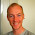## Monday, March 7, 2016

### Just some random 'art'

The initial name of this blog was 'random-digit' for some reason. I've always been fascinated by how randomness can lead to art.

On my new pet-project I've designed a mini processor to generate random images.

This is the gist:
• The processor has a basic set of registers (x,y,r,g,b) and optionally up-to N 'general purpose' registers ( r0... rN); all registers are floats.
• The code of the processor is a list of attributions in the format <register> = <expression>.
• The <expressions> are binary trees with the nodes containing one operator ( + , - , / or  * ) and the leafs are any of: any register, a constant value X, Pi, sin(<expression>), cos(<expression>), sqrt(<expression>) or simply <expression>.
• The number of such attributions is configurable. E.g. here's an example of 16 attributions code:
•     y = r2 - r0
y = x * y
r1 = r / y
r = x - y
r2 = b * r2
x = g * sin(x + 0)
r2 = x - 0.30149573
g = r1 / b
x = r1 + r2
r0 = r2 - r2
g = r0 / g
g = PI - r0
r = r0 + y
g = r + r0
g = r + cos(cos((r1 + 0.9902641) - b) - r)
r = r - 0
r0 = b + g
y = 0 * x
r1 = x - g
y = b + r2
r = g / r0
x = r0 * x
r2 = y / r2
y = g + y
y = b * r2
r0 = cos(g + PI) / x
x = r0 - y
b = r2 + 0
r2 = y / g
y = r * y

The 'emulator' will then iterate over the randomly generated code and at the end of each full  execution will place a pixel of color (r,g,b) on position (x,y) and then resume from start. The absolute and modulus 1 of the registers  (val = abs(r) %1f) is applied to ensure the output value is within the image and a valid color.

Naturally most of the "software" is trash and is automatically discarded. From the rest, I manually discard many it for being too boring, and on occasion some is kept because 'it looks nice'. :)

Here's a couple of those:

#### 1 comment:

1.Nice. I do similar things with an Arduino and the LCD shield.